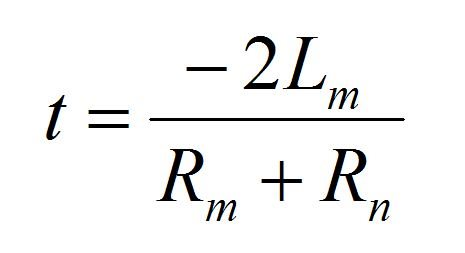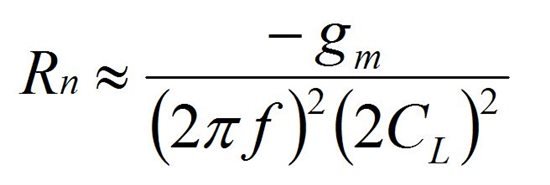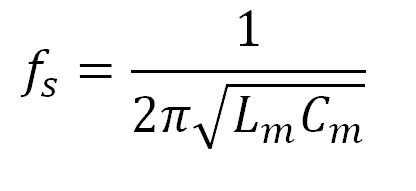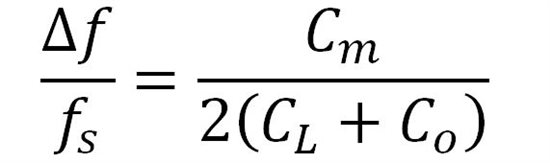# Frequency references: Startup time

In the previous post in this series, we discussed the crystal parameters including load capacitance CL, motional inductance Lm and motional capacitance Cm.  Continuing on, let’s suppose you have a choice between two 32MHz crystals, one with CL=10pF and one with C­L=16pF, and all other parameters are the same.  According to the SimpleLink™ Bluetooth low energy CC2540 solution specification, both crystals are supported, so which should you choose?  Well, it depends.

Crystal oscillator startup time approximately 7-15t, where t is:where Lm and Rm are the motional inductance and resistance parameters we’ve already seen and Rn is the negative resistance of the oscillator.The parameter gm is the oscillator transconductance, f is the crystal resonance frequency, and CL is the load capacitance.  From this equation, it is clear that a crystal with a lower CL is going to have a faster startup time.  This may save you power if you have a duty-cycled application because your system can spend more time in either sleep mode or receiving and transmitting and less time waiting for the reference clock to turn on.  Also, lower CL means that the negative resistance provided by the oscillator transistor is higher so that the oscillation can be sustained with lower power.  Given these power advantages, why would anyone choose a higher CL crystal?  The answer is frequency stability.  The unloaded oscillator frequency fs is shown below:The oscillator frequency shift as a function of the load capacitance is given by the following equation:Here you can see that if parasitic capacitance changes, which can be modeled as part of Co, the resulting frequency change will be less if CL is large.  If a crystal with a low CL is used, small changes in parasitics will cause larger changes in frequency.  In summary, choosing the crystal load capacitance is a tradeoff between power consumption and frequency stability.  Further information about choosing load capacitance value is available in the MSP430™ 32kHz crystal oscillation application note.

Stop by the blog in the coming weeks for part four of my series where I will discuss more considerations for choosing a crystal.

And if you missed the first two parts of this series, check them out below!

‒      Part 1: Frequency references 101